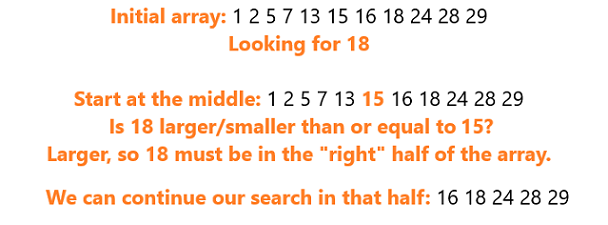# Binary Search in JavaScriptIn this article, we'll look at the idea behind Binary Search and how to implement it in JavaScript. Binary Search is a very simple, intuitive, yet efficient searching algorithm. The only caveat is that it works only sorted arrays, so some preprocessing on our data in order to sort it might be necessary.

#### Introduction

Searching is one of the most commonly performed tasks in the domain of Computer Science. Many algorithms and data structures exist to make searching more efficient.

In this article, we'll look at the idea behind Binary Search and how to implement it in JavaScript.

Binary Search is a very simple, intuitive, yet efficient searching algorithm. The only caveat is that it works only sorted arrays, so some preprocessing on our data in order to sort it might be necessary.

Binary Search is a divide-and-conquer algorithm, that divides the array roughly in half every time it checks whether an element of the array is the one we're looking for.

In other words, we divide the problem into simpler problems until it becomes simple enough to solve them directly. Let's assume we have a sorted array (in ascending order) and take a look at the steps of binary search:

1. Find the middle element of the given array.
2. Compare the middle element with the value we are looking for (called key).
• If the key is less than the middle element, search in the left half.
• If the key is more than the middle element, search in the right half.
• If the key is equal to the middle element, return the index of the middle element.
1. Continue with steps 1, 2 until we are left with a single element.

## Algorithms with JavaScript

A Day with JavaScript Algorithms.

## The essential JavaScript concepts that you should understand

The essential JavaScript concepts that you should understand - For successful developing and to pass a work interview

## quicksort Algorithmic in JavaScript

quicksort Algorithmic in JavaScript explain in js

## Data Types In JavaScript

JavaScript data types are kept easy. While JavaScript data types are mostly similar to other programming languages; some of its data types can be unique. Here, we’ll outline the data types of JavaScript.

## Binary Search Algorithm in Javascript

In this article, we will talk about binary search algorithm in Javascript. For today’s algorithm, I picked the binary search which is one of the most commonly asked topics in technical interviews. The problem named binary search from LeetCode will be used to explain this concept: